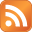» IT tipps and howto's

Shell scripting: if test [] examples

Last Update: 12.11.2009

Here you may find several examples of test constructs.

Important operators (for integers) to keep in mind:
-eq equal to
-ne not equal to
-lt less than
-le less than or equal to
-gt greater than
-ge greater than or equal to

If you test the existance of files:
-s file exists and is not empty
-f file exists and is not a directory
-d directory exists
-x file is executable
-w file is writable
-r file is readable

# Compares the values of two variables
if [ \${value1} = \${value2} ]
then echo "Both variables have the same value"
else echo "The variables values differ!"
fi

# Checks if a variable is NULL or not (#1 with -n and quotes)
if [ -n "\${var}" ]
then echo "The variable is not empty (not null)"
else echo "The variable is NULL"
fi

# Checks if a variable is NULL or not (#2)
if [ \${var} ]
then echo "The variable is not empty (not null)"
else echo "The variable is NULL"
fi

# Checks if a variable is bigger than a number (INTEGER)
if [ \${var} > 2 ]
then echo "The variable is bigger than two"
else echo "The variable is smaller than two"
fi

# The previous test doesnt work if the integer is 0 so do this:
if [ \${var} -gt 0 ]
then echo "The variable is bigger than zero"
else echo "The variable is smaller than zero"
fi

# Combination of two tests (note the echo 'at least')
if [ \${var1} > 2 ] || [ \${var2} > 2 ]
then echo "At least one of both variables is bigger than two"
else echo "No variable is bigger than two"
fi

# Combination of two tests (note the echo 'both')
if [ \${var1} > 5 ] && [ \${var2} > 5 ]
then echo "Both variables are bigger than five"
else echo "Not both variables are bigger than five"
fi